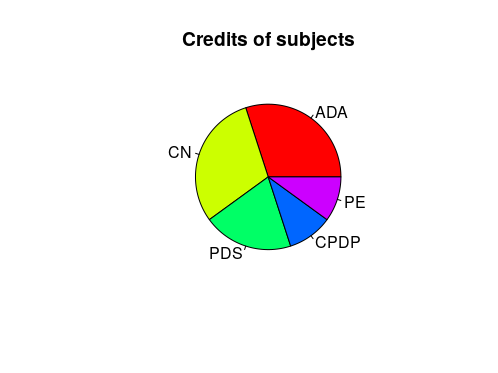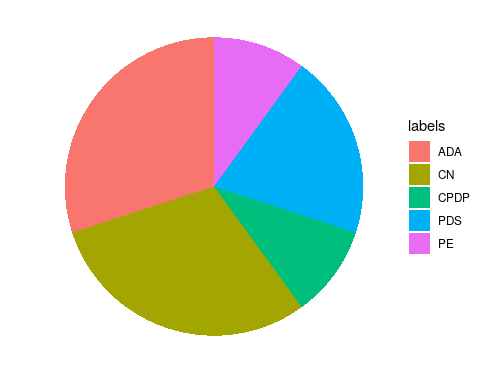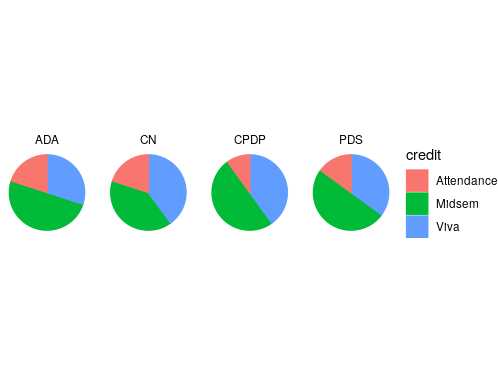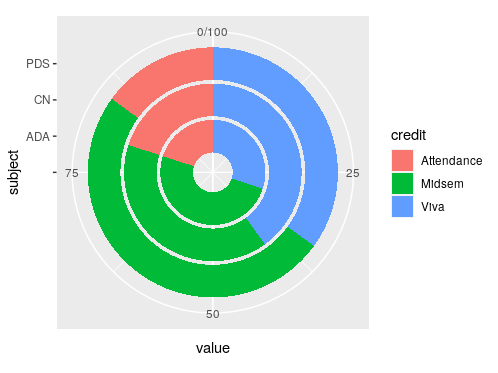Open In App

# Create Multiple Pie Charts using ggplot2 in R

Pie Chart, also known as circle chart, is the graphical representation of the relative size or frequency of the data in a circular format. Basically, it helps in visualizing the relative size or frequency of a particular group of data as a part of the whole. This article discusses how multiple pie charts can be created into one frame for consecutive comparison.

Function used:

• pie() function as the name suggests is used for visualizing a pie chart.

Syntax: pie(x, labels, radius, main, col, clockwise)

Parameters:

• x: This parameter is the vector containing the value of the pie chart.
• labels: This parameter is the vector containing the labels of all the slices in Pie Chart.
• radius: This parameter is the value of the radius of the pie chart. This value is between -1 to 1.
• main: This parameter is the title of the chart.
• col: This parameter is the color used in the pie chart.
• clockwise: This parameter is the logical value which is used to draw the slices in clockwise or anti-clockwise direction.
• coord_polar() function is used to create a polar coordinate system, which helps in drawing a pie chart.

Syntax:

coord_polar(theta = “x”, start = 0, direction = 1, clip = “on”)

Parameter:

• theta represents the angle
• start used for setting offset
• direction
• clip decides whether drawing should be clipped or not
• facet_grid() creates a matrix to display rows and columns faceting variables

Syntax:

facet_grid(facets, margins=FALSE, scales=”fixed”, space=”fixed”, shrink=TRUE, labeller=”label_value”, as.table=TRUE, drop=TRUE)

Let us first create a regular pie chart

Program 1 : Regular Pie Chart

## R

 `x <- ``c``(3,3,2,1,1)`` ` `labels <- ``c``(``'ADA'``,``'CN'``,``'PDS'``,``'CPDP'``,``'PE'``)`` ` `pie``(x, labels, main=``"Credits of subjects"``, col=``rainbow``(``length``(x)))`

Output:For building a Pie Chart in R, we can use ggplot2 package, but it does not have a direct method to do so. Instead, we plot a bar graph and then convert it into Pie Chart using coord_polar() function.

Approach:

• Import library
• Create data
• Create dataframe
• Plot a bar graph
• Convert bar graph into Pie chart
• Remove numerical values and grid

Program 2: Pie Chart using ggplot2

## R

 `library``(ggplot2)`` ` `df = ``data.frame``(x <- ``c``(3,3,2,1,1),``                ``labels <- ``c``(``'ADA'``,``'CN'``,``'PDS'``,``'CPDP'``,``'PE'``))`` ` `ggplot``(df, ``aes``(x=``""``, y=x, fill=labels)) +``geom_bar``(width = 1, stat = ``"identity"``) +``  ``coord_polar``(``"y"``, start=0) +``theme_void``()`

Output:To plot multiple pie charts in R using ggplot2, we have to use an additional method named facet_grid(). This method forms a matrix defined by row and column faceting variables. When we have two different variables and need a matrix with all combinations of these two variables, we use this method.

Approach:

• Import library
• Create dataframe
• Convert variables into categorical variables
• Plot Bar graph
• Convert into Pie Chart

Program 3: Multiple Pie Chart

## R

 `library``(ggplot2)``df = ``data.frame``(subject <- ``c``(``'ADA'``,``'ADA'``,``'ADA'``,``'CN'``,``'CN'``,``'CN'``,``'PDS'``,``'PDS'``,``'PDS'``,``'CPDP'``,``                         ``'CPDP'``,``'CPDP'``),``                ``credit <- ``c``(``'Midsem'``,``'Viva'``,``'Attendance'``,``'Midsem'``,``'Viva'``,``'Attendance'``,``                            ``'Midsem'``,``'Viva'``,``'Attendance'``,``'Midsem'``,``'Viva'``,``'Attendance'``),``                ``value <- ``c``(50,30,20,40,40,20,50,35,15,50,40,10))`` ` `df\$subject <- ``factor``(df\$subject)``df\$credit <- ``factor``(df\$credit) `` ` `ggplot``(data=df, ``aes``(x=``" "``, y=value, group=credit, colour=credit, fill=credit)) +``         ``geom_bar``(width = 1, stat = ``"identity"``) +``         ``coord_polar``(``"y"``, start=0) + ``         ``facet_grid``(.~ subject) +``theme_void``()`

Output:We can also plot multiple pie charts in the form of a donut chart using ggplot2 in R.

Approach:

• Import library
• Create dataframe
• Convert variables into categorical variables
• Plot Bar graph using geom_col()
• Add an empty element before the subjects using scale_x_discrete()
• Convert into Pie Chart using coord_polar()

Program 4: Multiple Pie Chart/ Donut Chart

## R

 `library``(ggplot2)``df = ``data.frame``(subject <- ``c``(``'ADA'``,``'ADA'``,``'ADA'``,``'CN'``,``'CN'``,``'CN'``,``'PDS'``,``'PDS'``,``'PDS'``),``                ``credit <- ``c``(``'Midsem'``,``'Viva'``,``'Attendance'``,``'Midsem'``,``'Viva'``,``'Attendance'``,``                            ``'Midsem'``,``'Viva'``,``'Attendance'``),``                ``value <- ``c``(50,30,20,40,40,20,50,35,15))`` ` `df\$subject <- ``factor``(df\$subject) ``# converts to a categorical variable``df\$credit <- ``factor``(df\$credit) ``# converts to a categorical variable`` ` ` ` `ggplot``(df, ``aes``(x = subject, y = value, fill = credit)) +``  ``geom_col``() +``scale_x_discrete``(limits = ``c``(``" "``, ``"ADA"``,``"CN"``,``"PDS"``)) +``coord_polar``(``"y"``)`

Output: